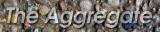# Numerical Precision, Accuracy, and Range

Computers do not process real numbers nor even integers. They process finite subsets of either and, unfortunately, operations on these finite values do not have exactly the same properties that most math classes teach. Generally, the numeric types used are identified primarily by their fixed precision: how many bits hold each value? However, accuracy is generally far more important: how close is the value to the value that would have been obtained using infinite precision? The relationship between accuracy and precision is disturbingly subtle.

For example, given finite-precision floating-pont values, (a+b)+c often yields a very different value from a+(b+c). Minor restructuring of the operation sequence can yield single precision results that are more accurate than those originally obtained using double precision! Even using integers, there are suprizes; for example, averaging two values, a and b, is not as simple as (a+b)/2 nor even (a/2)+(b/2) (here is a way to get the accurate floor of the average). There is an old joke that it is easy for a computer to do arithmetic very fast, so long as the answer doesn't have to be correct... we aren't laughing. Instead, we've been doing a lot toward making accuracy as predictable and controllable as possible with minimal computational overhead:

• Floating-Point Computation with Just Enough Accuracy (local copy) discusses and microbenchmarks native pair floating-point arithmetic optimized for various DSP, SWAR, and GPU targets; it also introduces the base concepts of speculative precision. This paper has been published in Lecture Notes in Computer Science, Volume 3991/2006, ISSN: 0302-9743, pp. 226-233; it also will be presented at Computational Science ICCS 2006: 6th International Conference, Reading, UK, May 28-31, 2006.
```@article{FPCwJEA,
author={Hank Dietz and Bill Dieter and Randy Fisher and Kungyen Chang},
title={Floating-Point Computation with Just Enough Accuracy},
journal={Lecture Notes in Computer Science},
volume={3991},
month={Apr},
year={2006},
pages={226 -- 233},
URL={http://dx.doi.org/10.1007/11758501_34}
}```
• Low-Cost Microarchitecture Support for Improved Floating-Point Accuracy describes some hardware extensions that help reduce the cost of doing native-pair floating-point operations. This paper has been published in Computer Architecture Letters. The paper references this technical report, which is a longer version of the paper, also discussing speculative precision.
```@Article{DiKD07,
author = 	 {William R. Dieter and Akil Kaveti and Henry G. Dietz},
title = 	 {Low-Cost Microarchitectural Support for Improved
Floating-Point Accuracy},
journal = 	 {IEEE Computer Architecture Letters},
year = 	 {2007},
volume = 	 {6},
number = 	 {1},
month = 	 {March},
url = {ieeexplore.ieee.org/xpls/pre_abs_all.jsp?isnumber=32572&arnumber=101109LCA20071},
}

@TechReport{dd06a,
author = 	 {William R. Dieter and Henry G. Dietz},
title = 	 {Low-Cost Microarchitectural Support for Improved Floating-Point Accuracy},
institution =  {University of Kentucky},
year = 	 {2006},
number = 	 {ECE-2006-10-14},
address = 	 {Electrical and Computer Engineering Dept., University of Kentucky, Lexington, KY 40506-0046, {\tt http://www.engr.uky.edu/~dieter/pub/TR-ECE-2006-10-14}},
month = 	 {October},
url = 	 {http://aggregate.org/NPAR/TR-ECE-2006-10-14.pdf}
}```
• The Magic Algorithms page gives a few relevant algorithms
• Explicit specification of bit-level precision and range (including saturation types) is implemented in both the SWARC and BitC languages, along with highly efficient codings

It is interesting to note that, just over the past year, GPUs have now standardized a new, even lower precision, floating-point format: EXT_packed_float values place 3 unsigned floating-point numbers in each 32-bit object. The RGB encoding uses a 5-bit exponent with a bias of -15. R and G each get a 6-bit mantissa, while B gets only 5 bits. That gives field sizes of 11, 11, and 10 bits. Here slide 16 gives a nice summary.The only thing set in stone is our name.